## UGC NET CS 2012 Dec-Paper-2

 Question 1
Consider the circuit shown below. In a certain steady state, Y is at logical ‘1’. What are possible values of A, B, C ?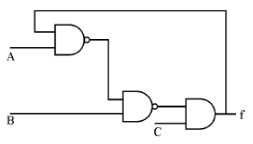A A = 0, B = 0, C = 1 B A = 0, B = C = 1 C A = 1, B = C = 0 D A = B = 1, C = 1 E A and D correct
Digital-Logic-Design       Logic-Gates
Question 1 Explanation:
The output f of the circuit is given as feedback input which should always be 1.
From the given circuit
f= (A+B')C (Assuming that feedback input is 1)
So, the output remains 1 iff C=1 and (A+B')=1.
A=1, B=0, C=1
A=1, B=1, C=1
A=0, B=0, C=1
Hence option A and D are correct.
 Question 2
The worst case time complexity of AVL tree is better in comparison to binary search tree for
 A Search and Insert Operations B Search and Delete Operations C Insert and Delete Operations D Search, Insert and Delete Operations
Data-Structures       Binary-Trees
Question 2 Explanation:
AVL tree is a self balancing tree with height difference at most 1. A balance factor in AVL tree is used to check what rotation to make.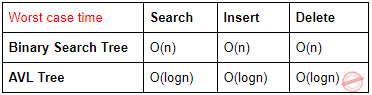Question 3
The GSM network is divided into the following three major systems :
 A SS, BSS, OSS B BSS, BSC, MSC C CELL, BSC, OSS D SS, CELL, MSC
Question 3 Explanation:
GSM Architecture:Mobile Station (MS)
Mobile Equipment (ME)
Subscriber Identity Module (SIM)
Base Station Subsystem (BSS)
Base Transceiver Station (BTS)
Base Station Controller (BSC)
Network Switching Subsystem (NSS)
Mobile Switching Center (MSC)
Home Location Register (HLR)
Visitor Location Register (VLR)
Authentication Center (AUC)
Equipment Identity Register (EIR)
 Question 4
The power set of the set {ɸ} is
 A {ɸ} B {ɸ, {ɸ}} C {0} D {0, ɸ, {ɸ}}
Engineering-Mathematics       Set-Theory
Question 4 Explanation:
The given set is not a null set don't get confused in this.
A null set is a set which have no element in it and can be represented as either { } or ɸ.
And the given set {ɸ} represents a set having ɸ as its element. It is not a null set because given set is having ɸ inside it.
As we know power set is a set of all subsets of a given set. For given set it's power set is {ɸ, {ɸ}}
 Question 5
If the disk head is located initially at 32, find the number of disk moves required with FCFS if the disk queue of I/O blocks requests are 98, 37, 14,124, 65, 67.
 A 239 B 310 C 321 D 325
Operating-Systems       Disk-scheduling
Question 5 Explanation: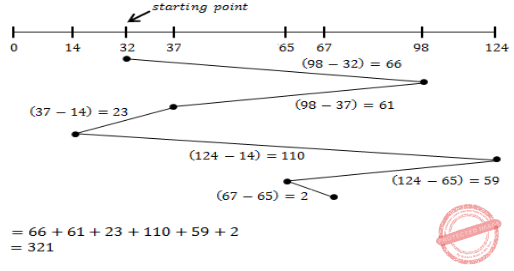Question 6
Component level design is concerned with
 A Flow oriented analysis B Class based analysis C Both of the above D None of the above
Question 6 Explanation:
Explanation:Question 7
The ‘C’ language is
 A Context free language B Context sensitive language C Regular language D None of the above
Theory-of-Computation       Languages-and-Grammars
Question 7 Explanation:
C and C++ are context sensitive languages.
 Question 8
The Mobile Application Protocol (MAP) typically runs on top of which protocol ?
 A SNMP (Simple Network Management Protocol) B SMTP (Simple Mail Transfer Protocol) C SS7 (Signalling System 7) D HTTP (Hypertext Transfer Protocol)
Computer-Networks       Application-Layer-Protocol
Question 8 Explanation:
→ The Mobile Application Protocol (MAP) is an SS7 protocol that provides an application layer for the various nodes in GSM and UMTS mobile core networks and GPRS core networks to communicate with each other in order to provide services to users.
→ The Mobile Application Protocol is the application-layer protocol used to access the Home Location Register, Visitor Location Register, Mobile Switching Center, Equipment Identity Register, Authentication Centre, Short message service center and Serving GPRS Support Node (SGSN).
 Question 9
If a packet arrive with an M-bit value is ‘1’ and a fragmentation offset value ‘0’, then it is ______ fragment.
 A First B Middle C Last D All of the above
Computer-Networks       IPv4-an-Fragmentation
Question 9 Explanation:
M bit is 1: It is either the first fragment or a middle one because of the offset value is 0, it is the first fragment.
M bit is 0: It means there are no more fragments. The fragment is the last one.
 Question 10
The number of bit strings of length eight that will either start with a 1 bit or end with two bits 00 shall be
 A 32 B 64 C 128 D 160
Engineering-Mathematics       Combinatorics
Question 10 Explanation:
→ Number of bit strings of length 8 that start with 1: 27 = 128.
→ Number of bit strings of length 8 that end with 00: 26 = 64.
→ Number of bit strings of length 8 that start with 1 and end with 00: 25 = 32.
→ Applying the subtraction rule, the number is 128+64−32 = 160
 Question 11
In compiler design ‘reducing the strength’ refers to
 A reducing the range of values of input variables. B code optimization using cheaper machine instructions. C reducing efficiency of program. D None of the above
Compiler-Design       Compilers
Question 11 Explanation:
In compiler design ‘reducing the strength’ refers to code optimization using cheaper machine instructions.
Example:
Dividing by 2→ Use right shift by 2.
Multiplication by 2→ Use left shift by 2.
 Question 12
In which addressing mode, the effective address of the operand is generated by adding a constant value to the contents of register ?
 A Absolute B Indirect C Immediate D Index
Question 12 Explanation:
1. An absolute address is represented by the contents of a register. This addressing mode is absolute in the sense that it is not specified relative to the current instruction address.
2. Indirect addressing is a scheme in which the address specifies which memory word or register contains not the operand but the address of the operand.
Immediate Operand:
The simplest way for an instruction to specify an operand is for the address part of the instruction actually to contain the operand itself rather than an address or other information describing where the operand is. Such an operand is called an immediate operand because it is automatically fetched from memory at the same time the instruction itself is fetched. It is immediately available for use.
Index mode:
The address of the operand is obtained by adding to the contents of the general register (called index register) a constant value. The number of the index register and the constant value are included in the instruction code
 Question 13
Which of the following is true ?
 A A relation in BCNF is always in 3NF. B A relation in 3NF is always in BCNF. C BCNF and 3NF are same. D A relation in BCNF is not in 3NF.
Question 13 Explanation:
Explanation:Question 14
Given memory partitions of 100 K, 500 K, 200 K, 300 K and 600 K (in order) and processes of 212 K, 417 K,112 K, and 426 K (in order), using the first-fit algorithm, in which partition would the process requiring 426 K be placed ?
 A 500 K B 200 K C 300 K D 600 K E None of the above
Operating-Systems       Memory-Management
Question 14 Explanation:
First fit: Allocate the first hole that is big enough. Searching can start either at the beginning of the set of holes or at the location where the previous first-fit search ended. We can stop searching as soon as we find a free hole that is large enough.Note: Given options are wrong. Excluded for evaluation.
 Question 15
What is the size of the Unicode character in Windows Operating System ?
 A 8-Bits B 16-Bits C 32-Bits D 64-Bits
Operating-Systems       Windows-Operating-System
Question 15 Explanation:
→ The most common encoding of Unicode and the one used for native Unicode encoding on Windows operating systems.
→ Each code value is 16 bits wide, in contrast to the older code page approach to character and string data, which uses 8-bit code values.
→ The use of 16 bits allows the direct encoding of 65,536 characters.
 Question 16
In which tree, for every node the height of its left subtree and right subtree differ almost by one ?
 A Binary search tree B AVL tree C Threaded Binary Tree D Complete Binary Tree
Data-Structures       Binary-Trees
Question 16 Explanation:
→ AVL tree is a self balancing tree with height difference at most 1. A balance factor in AVL tree is used to check what rotation to make.
→ ALV tree
 Question 17
The design issue of Data link Layer in OSI Reference Model is
 A Framing B Representation of bits C Synchronization of bits D Connection control
Question 17 Explanation:
Explanation:Question 18
Given the following expressions of a grammar
E → E * F / F + E / F
F → F – F / id
Which of the following is true ?
 A * has higher precedence than + B – has higher precedence than * C + and – have same precedence D + has higher precedence than *
Compiler-Design       Grammars
Question 18 Explanation:
The operator which is in low level that can have high preference.
Order of precedence is *, +, -.
Here * and + have equal preference, '-' can have higher precedence than + and *.
 Question 19
The maturity levels used to measure a process are
 A Initial, Repeatable, Defined, Managed, Optimized. B Primary, Secondary, Defined, Managed, Optimized. C Initial, Stating, Defined, Managed, Optimized. D None of the above
Question 19 Explanation:
CMM stands for Capability Maturity Model is a process model which specifies the process improvement approach in software development.
CMM levels: →Initial
→Repeatable
→Defined
→Managed
→Optimizing
 Question 20
The problem of indefinite blockage of low-priority jobs in general priority scheduling algorithm can be solved using :
 A Parity bit B Aging C Compaction D Timer
Operating-Systems       CPU-Scheduling
Question 20 Explanation:
→ Ageing is a scheduling technique used to avoid starvation (or) indefinite blocking.
→ A task with a high priority is allowed to access a specific system resource before a task with a lower priority is allowed to do the same.
→ A disadvantage of this approach is that tasks assigned with a lower priority may be starved when a large number of high priority tasks are queued.
→ Aging is used to gradually increase the priority of a task, based on its waiting time in the ready queue.
 Question 21
Which API is used to draw a circle ?
 A Circle( ) B Ellipse( ) C Round Rect( ) D Pie( )
Question 21 Explanation:
→ When we are giving same height and width to a ellipse it will become circle.
→ API is a protocol intended to be used as an interface by software components to communicate with each other.
→ An API is a library that may include specification for routines, data structures, object classes and variables
 Question 22
In DML, RECONNCT command cannot be used with
 A OPTIONAL Set B FIXED Set C MANDATOR Set D All of the above
Database-Management-System       SQL
Question 22 Explanation:
→ The RECONNECT command can be used with both OPTIONAL and MANDATORY sets, but not with FIXED sets.
→ The RECONNECT command moves a member record from one set instance to another set instance of the same set type. It cannot be used with FIXED sets because a member record cannot be moved from one set instance to another under the FIXED constraint.
 Question 23
Coaxial cables are categorized by Radio Government rating are adapted for specialized functions. Category RG-59 with impedance 75Ω used for
 A Cable TV B Ethernet C Thin Ethernet D Thick Ethernet
Computer-Networks       Ethernet
Question 23 Explanation:
RG-59/U with impedance 75Ω used to carry baseband video in closed-circuit television, previously used for cable television. In general, it has poor shielding but will carry an HQ HD signal or video over short distances.
RG-59/AU with impedance 75Ω is similar physical characteristics as RG-59 and RG-59/U, but with a higher velocity factor. 8.9@700 MHz
 Question 24
RAD stands for ______.
 A Rapid and Design B Rapid Aided Development C Rapid Application Development D Rapid Application Design
Question 24 Explanation:
→ Rapid Application Development(RAD) is used when the requirements cannot or will not be specified.
Rapid Application Development refers to a software development approach which puts less emphasis on planning and even design specifications, and more on rapid development and quick feedback. Prototypes are rapidly delivered to users to ensure they’re directly involved in feedback and development. There are also tools for collecting data, designing personal workflows, and setting up unique rules for each new application.
 Question 25
Suppose that someone starts with a chain letter. Each person who receives the letter is asked to send it on to 4 other people. Some people do this, while some do not send any letter. How many people have seen the letter, including the first person, if no one receives more than one letter and if the chain letter ends after there have been 100 people who read it but did not send it out ? Also find how many people sent out the letter ?
 A 122 & 22 B 111 & 11 C 133 & 33 D 144 & 44
Engineering-Mathematics       Combinatorics
Question 25 Explanation:
→ Notice that since every person who sends out the letter sends it to exactly 4 other people, and no two people receive the letter twice, this situation can be modeled using a full rooted 4-ary tree.
→ The root represents the person who first sends out the letter, and the children of any vertex represent the 4 people that the related person sent letters.
→ From the question, total people are 100, The total number of people who received the letter can be found by computing the number total number of vertices in the rooted tree.
3n+1=100
n=100-⅓
=33
The number of people who read it but did not send it out is 100+33=133
The number of people sent out the letter is 33
 Question 26
A hash function f defined as f(key) = key mod 13, with linear probing is used to insert keys 55, 58, 68, 91, 27, 145. What will be the location of 79 ?
 A 1 B 2 C 3 D 4
Data-Structures       Hashing
Question 26 Explanation:Question 27
Which of the following is true while converting CFG to LL(I) grammar ?
 A Remove left recursion alone B Factoring grammar alone C Both of the above D None of the above
Compiler-Design       Parsers
Question 27 Explanation:
→ A grammar could be LL(1) if and only if it is unambiguous and is not left recursive and left factoring grammar.
→ Since for converting a grammar to LL(1) 3 conditions mentioned above are required.
→ Unambiguous grammar is not mentioned in options. So option (D) is the correct.
 Question 28
Identify the Risk factors which are associated with Electronic payment system.
 A Fraudulent use of Credit Cards. B Sending Credit Card details over internet. C Remote storage of Credit Card details. D All of the above
Question 28 Explanation:
Electronic payment system risk factors: →Fraudulent use of Credit Cards.
→Sending Credit Card details over internet.
→Remote storage of Credit Card details.
 Question 29
Which of the following are two special functions that are meant for handling exception, that occur during exception handling itself ?
 A Void terminate ( ) and Void unexpected ( ) B Non void terminate ( ) and void unexpected ( ) C Void terminate ( ) and non void unexpected ( ) D Non void terminate ( ) and non void unexpected ( )
Programming-in-c++       Properties
 Question 30
Which of the following memory allocation scheme suffers from external fragmentation ?
 A Segmentation B Pure demand paging C Swapping D Paging
Operating-Systems       Memory-Management
 Question 31
Basis path testing falls under
 A system testing B white box testing C white box testing D unit testing
Software-Engineering       Software-testing
Question 31 Explanation:
White-box test design techniques include the following code coverage criteria: →Control flow testing
→Data flow testing
→Branch testing
→Statement coverage
→Decision coverage
→Modified condition/decision coverage
→Prime path testing
→Path testing
 Question 32
The User Work Area (UWA) is a set of Program variables declared in the host program to communicate the contents of individual records between
 A DBMS & the Host record B Host program and Host record C Host program and DBMS D Host program and Host language
Question 32 Explanation:
→ A set of program variables, declared in the host program, to communicate the contents of individual records between the DBMX and the host program.
→ For each record type in the database schema, a corresponding program variable with the same format should be declared in the host program.
 Question 33
Consider the tree given below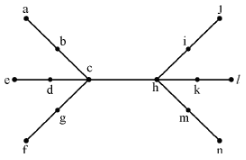Using the property of eccentricity of a vertex, find every vertex that is the centre of the given tree.
 A d & h B c & k C g, b, c, h, i, m D c & h
Data-Structures       Binary-Trees
Question 33 Explanation: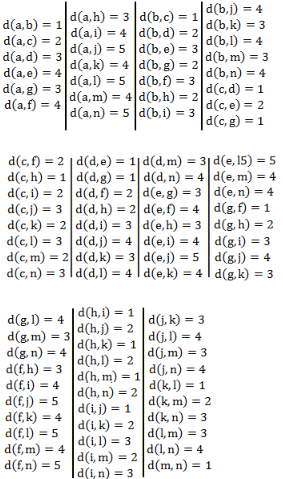Eccentricity of a vertex u, is
E(u) = maxu∈v(G) d(u, v)
i.e., the maximum distance of a node to other nodes is the eccentricity of that node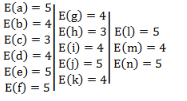Radius: The minimum eccentricity from all the vertices is considered as the radius of the Graph G denoted by r(G).
Here radius of the graph, r(G)=3
Center:- If the eccentricity of a graph is equal to its radius, then it is known as the central point of the graph.
And in above graph vertex "h" and " c" have eccentricity equal to the radius of the graph. So vertex h and c are the center of graph.
Hence correct answer is option (D).
 Question 34
The maximum number of keys stored in a B-tree of order m and depth d is
 A md+1–1 B (md+1–1) / (m–1) C (m–1) (md+1–1) D (md–1) / (m–1) E None of the above
Database-Management-System       B-and-B+-Trees
Question 34 Explanation:
Order of a B-tree represents the number of children a node can have and we know the number of keys is always equals to the (Order of B-tree) - 1So here each of the ‘m’ children is having (m-1) keys.
Hence total no. of keys = m1 (m-1) keys
(3) Similarly, for a B-tree of order ‘d’ total no. of keys possible = md (m-1) keys
 Question 35
Which of the following is the most powerful parsing method ?
 A LL(I) B Canonical LR C SLR D LALR
Compiler-Design       Parsers
Question 35 Explanation:
Canonical LR is most powerful.
LR > LALR > SLR.
But real time compilers using LALR only
 Question 36
In UNIX, which of the following command is used to set the task priority ?
 A init B nice C kill D PS
Operating-Systems       UNIX-Operating-System
Question 36 Explanation:
init (short for initialization) is the first process started during booting of the computer system. Init is a daemon process that continues running until the system is shut down. It is the direct or indirect ancestor of all other processes and automatically adopts all orphaned processes. Init is started by the kernel during the booting process; a kernel panic will occur if the kernel is unable to start it. Init is typically assigned process identifier 1.
nice directly maps to a kernel call of the same name. nice is used to invoke a utility or shell script with a particular priority, thus giving the process more or less CPU time than other processes. A niceness of −20 is the highest priority and 19 is the lowest priority. The default niceness for processes is inherited from its parent process and is usually 0.
kill It will kill the process
ps (short for "process status") displays the currently-running processes.
 Question 37
AES is a round cipher based on the Rijndael Algorithm that uses a 128-bit block of data. AES has three different configurations. ______ rounds with a key size of 128 bits, ______ rounds with a key size of 192 bits and ______ rounds with a key size of 256 bits.
 A 5, 7, 15 B 10, 12, 14 C 5, 6, 7 D 20, 12, 14
Computer-Networks       Network-Security
Question 37 Explanation:
→ AES is a round cipher based on the Rijndael Algorithm that uses a 128-bit block of data.
→ The key size used for an AES cipher specifies the number of transformation rounds that convert the input, called the plaintext, into the final output, called the ciphertext.
→ The number of rounds are as follows:
1. 10 rounds with a key size of 128 bits.
2. 12 rounds with a key size of 192 bits.
3. 14 rounds with a key size of 256 bits.
 Question 38
Match the following IC families with their basic circuits :A a-1, b-2, c-3 B a-3, b-2, c-1 C a-2, b-3, c-1 D a-2, b-1, c-3
Question 38 Explanation:
Transistor Transistor Logic(TTL) → NAND
Emitter Coupled Logic(ECL) → NOR
Complementary Metal Oxide Semiconductor(CMOS) → Inverter
 Question 39
Match the following with respect to C++ data types :A a-2, b-3, c-4, d-1 B a-3, b-1, c-4, d-2 C a-4, b-1, c-2, d-3 D a-3, b-4, c-1, d-2
Programming-in-c++       Data-Types
Question 39 Explanation:
User defined type→ Union
Built in type→ Void
Derived type→ Pointer
Long double→ Qualifier
 Question 40
Given an empty stack, after performing Push(1), Push(2), Pop, Push(3), Push(4), Pop, Pop, push(5), Pop. what is the value of the top of the stack ?
 A 4 B 3 C 2 D 1
Data-Structures       Queues-and-Stacks
Question 40 Explanation: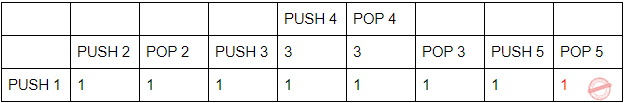Question 41
Enumeration is a process of
 A Declaring a set of numbers B Sorting a list of strings C Assigning a legal values possible for a variable D Sequencing a list of operators
Programming       Data-Type
Question 41 Explanation:
→ Enumeration is a data type consisting of a set of named values called elements, members, enumeral, or enumerators of the type.
→ Enumeration is a process of assigning a legal values possible for a variable
 Question 42
Which of the following mode declaration is used in C++ to open a file for input ?
 A ios : : app B in : : ios C ios : : file D ios : : in
Programming-in-c++       Declaration
Question 42 Explanation:
ios::in → Open a file for reading.
ios::app → Append mode. All output to that file to be appended to the end.
 Question 43
Data Encryption Techniques are particularly used for ______.
 A protecting data in Data Communication System. B reduce Storage Space Requirement. C enhances Data Integrity. D decreases Data Integrity.
Computer-Networks       Network-Security
Question 43 Explanation:
Data Encryption Techniques are particularly used for protecting data in Data Communication System.
 Question 44
Let L be a set accepted by a nondeterministic finite automaton. The number of states in non-deterministic finite automaton is |Q|. The maximum number of states in equivalent finite automaton that accepts L is
 A |Q| B 2|Q| C 2|Q| – 1 D 2|Q|
Theory-of-Computation       Finite-Automata
Question 44 Explanation:
The maximum number of states possible in a finite automaton which is equivalent to given non-deterministic finite automaton having with |Q| states is 2^|Q|
 Question 45
What is the result of the following expression? (1 & 2) + (3 & 4)
 A 1 B 3 C 2 D 0
Programming       Operator
Question 45 Explanation:
Given statement, (1&2)+(3/4)
(1&2) means (1 AND 2)
(¾) means (3 divided by 4)
Step-1: First consider (1&2)Question 46
Back propagation is a learning technique that adjusts weights in the neural network by propagating weight changes.
 A Forward from source to sink B Backward from sink to source C Forward from source to hidden nodes D Backward from since to hidden nodes
Question 46 Explanation:
→ Back propagation is a learning technique that adjusts weights in the neural network by propagating weight changes backward from sink to source.
→ Backpropagation is shorthand for "the backward propagation of errors," since an error is computed at the output and distributed backwards throughout the network’s layers.
 Question 47
Match the following :A a-3, b-2, c-1 B a-1, b-2, c-3 C a-1, b-3, c-2 D a-3, b-1, c-2
Question 47 Explanation:
Emitter Coupled Logic (ECL)
The storage time is eliminated as the transistors are used in difference amplifier mode and are never driven into saturation.
1. Fastest among all logic families
2. Lowest propagation delay.
Complementary metal oxide semiconductor(CMOS)
The power dissipation is usually 10nW per gate depending upon the power supply voltage, output load etc.
1. Lowest power dissipation
2. Excellent noise immunity
3. High packing density
4. Wide range of supply voltage
5. Highest fan out among all logic families
Transistor Transistor Logic:
→ TTL devices consume substantially more power than equivalent CMOS devices at rest, but power consumption does not increase with clock speed as rapidly as for CMOS devices.
 Question 48
______ is an “umbrella” activity that is applied throughout the software engineering process.
 A Debugging B Testing C Designing D Software quality assurance
Software-Engineering       Software-design
Question 48 Explanation:
Umbrella Activities 1. Software project tracking and control 2. Risk Management 3. Software Quality Assurance 4. Technical review 5. Software Configuration management 6. Reusability management 7. Work Product preparation and production
 Question 49
Identify the operation which is commutative but not associative ?
 A OR B NOR C EX-OR D NAND E None of the above
Digital-Logic-Design       Boolean-Operator
Question 49 Explanation:
OR is associative:
(i) (0 OR 1)OR 1
= 1 OR 1
= 1
(ii). 0 OR (1 OR 1)
= 0 OR 1
= 1
NAND is not associative
(i). (0 NAND 1)NAND 1
= 1 NAND 1
= 0
(ii). 0 NAND (1 NAND 1)
= 0 NAND 0
= 1
Ex-OR is associative
(i). (0 XOR 1) XOR 1
= 1 XOR 1
= 0
(ii). 0 XOR(1 XOR 1)
= 0 XOR 0
= 0
NOR is Not associative:
(i) (0 NOR 1) NOR 1
= 0 NOR 1
= 0
(ii). 0 NOR(1 NOR 1)
= 0 NOR 0
= 1
Note: Every logic gate is commutative.
 Question 50
Given a Relation POSITION (Posting- No, Skill), then query to retrieve all distinct pairs of posting-nos. requiring skill is
 A Select p.posting-No, p.posting No from position p where p.skill = p.skilland p.posting-No < p.posting-No B Select p1.posting-No, p2.posting- No from position p1, position p2 where p1.skill = p2.skill C Select p1.posting-No, p2posting-No from position p1, position p2 where p1.skill = p2.skill and p1.posting-No < p2.posting-No D Select p1.posting-No, p2.posting- No from position p1, position p2 where p1.skill = p2.skill and p1.posting-No = p2.posting-No
Database-Management-System       SQL
Question 50 Explanation:
Option (A) is incorrect because No self join is not possible without aliasing. Without aliasing it will through an error.
Option(B) is not correct because it is not resulting into distinct pairs of posting-nos.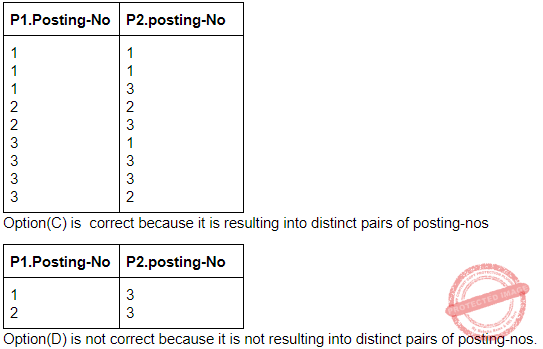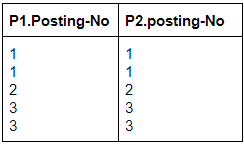There are 50 questions to complete.
PHP Code Snippets Powered By : XYZScripts.com